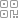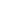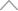6 2 9 1 1 1 8

# 原始数据都一样，为啥Pyecharts做出来的图一个是彩色的，另一个是黑白的？

## 一、思路

下面是他的代码，首先是读取excel文件，之后他用了两种方法生成数据，一个是datas，另外一个是datas2，这两个数据，最后通过比对，发现竟然是一样的，数据也都相等，但是唯独最后生成的html动图，有点不一样。

import pandas as pd

from pyecharts import options as opts

from pyecharts.charts import Map

import operator as op

import time

locations = [location for location in df_tb['地区']]

values = [value for value in df_tb['2016年']]

datas = list(zip(locations, values))

print(datas)

for data in datas:

print(data)

# print(type(data))

print(type(datas))

# print("==============================")

# def func(m):

#     a = []

#     for i in range(0, 35):

#         b = (df_tb['地区'][i], df_tb[m][i])

#         a.append(b)

#     return a

# datas2 = func('2016年')

# for data in datas2:

#     print(data)

#     print(type(data))

# print(datas2)

# print(type(datas2))

map = (

Map().

add('gdp', [location for location in datas], 'china')

# .add('gdp', [list(location) for location in datas], 'china')

.set_global_opts(

title_opts=opts.TitleOpts(title='各省贫困县分布图'),

visualmap_opts=opts.VisualMapOpts(max_=150)

)

)

map.render('各省贫困县分布图.html')

# print(op.eq(datas, func('2016年')))## 二、解决方法可以清晰的看到datas列表里边的数字的类型是int类型，而datas2列表里边的数字的类型是numpy.int64类型，而numpy.int64类型在html中是显示不出来的，因此问题就水落石出了。只需要在函数处理的时候将numpy.int64类型来个强转变为int类型，问题就迎刃而解了。只需要将func()函数中的代码替换成下面这个就可以了：

def func(m):

a = []

for i in range(0, 35):

b = (df_tb['地区'][i], int(df_tb[m][i]))

a.append(b)

return a## 新知精选

IT研发▪2021年度十佳创作者

### 优选课程 新知学院

•扫码下载商业新知APP~

•回到顶部